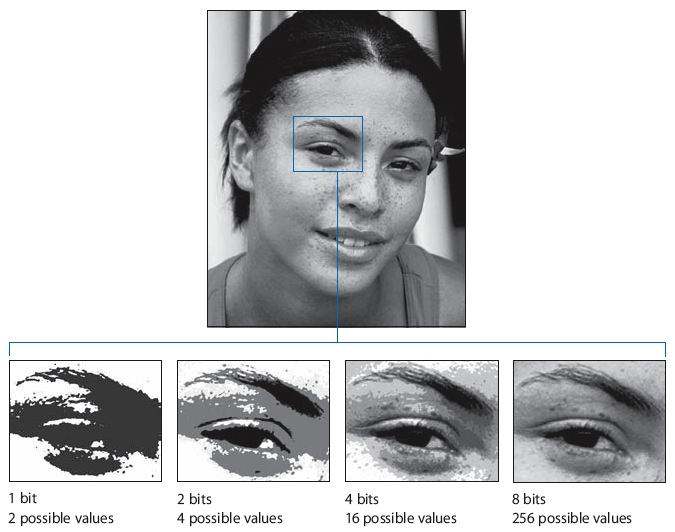### How bits work

You’ve probably heard before that computers store things in 1s and 0s. These fundamental units of information are known as bits. When a bit is “on,” it corresponds with a 1; when it’s “off,” it turns into a 0. Each bit, in other words, can store only two pieces of information.

But once you string them together, the amount of information you can encode grows exponentially. Two bits can represent four pieces of information because there are 2^2 combinations: 00, 01, 10, and 11. Four bits can represent 2^4, or 16 pieces of information. Eight bits can represent 2^8, or 256. And so on.

The right combination of bits can represent types of data like numbers, letters, and colors, or types of operations like addition, subtraction, and comparison. Most laptops these days are 32- or 64-bit computers. That doesn’t mean the computer can only encode 2^32 or 2^64 pieces of information total. (That would be a very wimpy computer.) It means that it can use that many bits of complexity to encode each piece of data or individual operation.

### 4-bit deep learning

So what does 4-bit training mean? Well, to start, we have a 4-bit computer, and thus 4 bits of complexity. One way to think about this: every single number we use during the training process has to be one of 16 whole numbers between -8 and 7, because these are the only numbers our computer can represent. That goes for the data points we feed into the neural network, the numbers we use to represent the neural network, and the intermediate numbers we need to store during training.

So how do we do this? Let’s first think about the training data. Imagine it’s a whole bunch of black-and-white images. Step one: we need to convert those images into numbers, so the computer can understand them. We do this by representing each pixel in terms of its grayscale value—0 for black, 1 for white, and the decimals between for the shades of gray. Our image is now a list of numbers ranging from 0 to 1. But in 4-bit land, we need it to range from -8 to 7. The trick here is to linearly scale our list of numbers, so 0 becomes -8 and 1 becomes 7, and the decimals map to the integers in the middle. So:

This process isn’t perfect. If you started with the number 0.3, say, you would end up with the scaled number -3.5. But our four bits can only represent whole numbers, so you have to round -3.5 to -4. You end up losing some of the gray shades, or so-called precision, in your image. You can see what that looks like in the image below.The lower the number of bits, the less detail the photo has. This is what is called a loss of precision.

This trick isn’t too shabby for the training data. But when we apply it again to the neural network itself, things get a bit more complicated.

We often see neural networks drawn as something with nodes and connections, like the image above. But to a computer, these also turn into a series of numbers. Each node has a so-called activation value, which usually ranges from 0 to 1, and each connection has a weight, which usually ranges from -1 to 1.

We could scale these in the same way we did with our pixels, but activations and weights also change with every round of training. For example, sometimes the activations range from 0.2 to 0.9 in one round and 0.1 to 0.7 in another. So the IBM group figured out a new trick back in 2018: to rescale those ranges to stretch between -8 and 7 in every round (as shown below), which effectively avoids losing too much precision.The IBM researchers rescale the activations and weights in the neural network for every round of training, to avoid losing too much precision.

But then we’re left with one final piece: how to represent in four bits the intermediate values that crop up during training. What’s challenging is that these values can span across several orders of magnitude, unlike the numbers we were handling for our images, weights, and activations. They can be tiny, like 0.001, or huge, like 1,000. Trying to linearly scale this to between -8 and 7 loses all the granularity at the tiny end of the scale.Linearly scaling numbers that span several orders of magnitude loses all the granularity at the tiny end of the scale. As you can see here, any numbers smaller than 100 would be scaled to -8 or -7. The lack of precision would hurt the final performance of the AI model.

After two years of research, the researchers finally cracked the puzzle: borrowing an existing idea from others, they scale these intermediate numbers logarithmically. To see what I mean, below is a logarithmic scale you might recognize, with a so-called “base” of 10, using only four bits of complexity. (The researchers instead use a base of 4, because trial and error showed that this worked best.) You can see how it lets you encode both tiny and large numbers within the bit constraints.